Main Content

## 1-D Decimated Wavelet Transforms

This section takes you through the features of 1-D critically-sampled wavelet analysis using the Wavelet Toolbox™ software.

The toolbox provides these functions for 1-D signal analysis. For more information, see the reference pages.

### Analysis-Decomposition Functions

Function Name

Purpose

`dwt`

Single-level decomposition

`wavedec`

Decomposition

`wmaxlev`

Maximum wavelet decomposition level

### Synthesis-Reconstruction Functions

Function Name

Purpose

`idwt`

Single-level reconstruction

`waverec`

Full reconstruction

`wrcoef`

Selective reconstruction

`upcoef`

Single reconstruction

### Decomposition Structure Utilities

Function Name

Purpose

`detcoef`

Extraction of detail coefficients

`appcoef`

Extraction of approximation coefficients

`upwlev`

Recomposition of decomposition structure

### Denoising and Compression

Function Name

Purpose

`wdenoise`

Automatic wavelet signal denoising (recommended)

`wdenoise2`

Automatic wavelet image denoising (recommended)

`ddencmp`

Provide default values for denoising and compression

`wbmpen`

Penalized threshold for wavelet 1-D or 2-D denoising

`wdcbm`

Thresholds for wavelet 1-D using Birgé-Massart strategy

`wdencmp`

Wavelet denoising and compression

`wden`

Automatic wavelet denoising

`wthrmngr`

Threshold settings manager

In this section, you'll learn how to

• Load a signal

• Perform a single-level wavelet decomposition of a signal

• Construct approximations and details from the coefficients

• Display the approximation and detail

• Regenerate a signal by inverse wavelet transform

• Perform a multilevel wavelet decomposition of a signal

• Extract approximation and detail coefficients

• Reconstruct the level 3 approximation

• Reconstruct the level 1, 2, and 3 details

• Display the results of a multilevel decomposition

• Reconstruct the original signal from the level 3 decomposition

• Remove noise from a signal

• Refine an analysis

• Compress a signal

• Show a signal's statistics and histograms

Since you can perform analyses either from the command line or using the Wavelet Analyzer app, this section has subsections covering each method.

The final subsection discusses how to exchange signal and coefficient information between the disk and the graphical tools.

### 1-D Analysis Using the Command Line

This example involves a real-world signal — electrical consumption measured over the course of 3 days. This signal is particularly interesting because of noise introduced when a defect developed in the monitoring equipment as the measurements were being made. Wavelet analysis effectively removes the noise.

Note

To denoise a signal, `wdenoise` and Wavelet Signal Denoiser are recommended.

1. Load the signal and select a portion for wavelet analysis.

```load leleccum; s = leleccum(1:3920); l_s = length(s);```
2. Perform a single-level wavelet decomposition of a signal.

Perform a single-level decomposition of the signal using the `db1` wavelet.

`[cA1,cD1] = dwt(s,'db1');`

This generates the coefficients of the level 1 approximation (`cA1`) and detail (`cD1`).

3. Construct approximations and details from the coefficients.

To construct the level 1 approximation and detail (`A1` and `D1`) from the coefficients `cA1` and `cD1`, type

```A1 = upcoef('a',cA1,'db1',1,l_s); D1 = upcoef('d',cD1,'db1',1,l_s);```

or

```A1 = idwt(cA1,[],'db1',l_s); D1 = idwt([],cD1,'db1',l_s);```
4. Display the approximation and detail.

To display the results of the level-one decomposition, type

```subplot(1,2,1); plot(A1); title('Approximation A1') subplot(1,2,2); plot(D1); title('Detail D1')```5. Regenerate a signal by using the Inverse Wavelet Transform.

To find the inverse transform, enter

```A0 = idwt(cA1,cD1,'db1',l_s); err = max(abs(s-A0))```
6. Perform a multilevel wavelet decomposition of a signal.

To perform a level 3 decomposition of the signal (again using the `db1` wavelet), type

`[C,L] = wavedec(s,3,'db1');`

The coefficients of all the components of a third-level decomposition (that is, the third-level approximation and the first three levels of detail) are returned concatenated into one vector, C. Vector L gives the lengths of each component.7. Extract approximation and detail coefficients.

To extract the level 3 approximation coefficients from `C`, type

```cA3 = appcoef(C,L,'db1',3); ```

To extract the levels 3, 2, and 1 detail coefficients from `C`, type

```cD3 = detcoef(C,L,3); cD2 = detcoef(C,L,2); cD1 = detcoef(C,L,1); ```

or

```[cD1,cD2,cD3] = detcoef(C,L,[1,2,3]); ```
8. Reconstruct the Level 3 approximation and the Level 1, 2, and 3 details.

To reconstruct the level 3 approximation from `C`, type

```A3 = wrcoef('a',C,L,'db1',3); ```

To reconstruct the details at levels 1, 2, and 3, from `C`, type

```D1 = wrcoef('d',C,L,'db1',1); D2 = wrcoef('d',C,L,'db1',2); D3 = wrcoef('d',C,L,'db1',3); ```
9. Display the results of a multilevel decomposition.

To display the results of the level 3 decomposition, type

```subplot(2,2,1); plot(A3); title('Approximation A3') subplot(2,2,2); plot(D1); title('Detail D1') subplot(2,2,3); plot(D2); title('Detail D2') subplot(2,2,4); plot(D3); title('Detail D3') ```10. Reconstruct the original signal from the Level 3 decomposition.

To reconstruct the original signal from the wavelet decomposition structure, type

```A0 = waverec(C,L,'db1'); err = max(abs(s-A0)) ```
11. Crude denoising of a signal.

Using wavelets to remove noise from a signal requires identifying which component or components contain the noise, and then reconstructing the signal without those components.

In this example, we note that successive approximations become less and less noisy as more and more high-frequency information is filtered out of the signal.

The level 3 approximation, `A3`, is quite clean as a comparison between it and the original signal.

To compare the approximation to the original signal, type

```subplot(2,1,1);plot(s);title('Original'); axis off subplot(2,1,2);plot(A3);title('Level 3 Approximation'); axis off ```Of course, in discarding all the high-frequency information, we've also lost many of the original signal's sharpest features.

Optimal denoising requires a more subtle approach called thresholding. This involves discarding only the portion of the details that exceeds a certain limit.

12. Remove noise by thresholding.

Let's look again at the details of our level 3 analysis.

To display the details `D1`, `D2`, and `D3`, type

```subplot(3,1,1); plot(D1); title('Detail Level 1'); axis off subplot(3,1,2); plot(D2); title('Detail Level 2'); axis off subplot(3,1,3); plot(D3); title('Detail Level 3'); axis off ```Most of the noise occurs in the latter part of the signal, where the details show their greatest activity. What if we limited the strength of the details by restricting their maximum values? This would have the effect of cutting back the noise while leaving the details unaffected through most of their durations. But there's a better way.

Note that `cD1`, `cD2`, and `cD3` are just MATLAB® vectors, so we could directly manipulate each vector, setting each element to some fraction of the vectors' peak or average value. Then we could reconstruct new detail signals `D1`, `D2`, and `D3` from the thresholded coefficients.

To denoise the signal, use the `ddencmp` command to calculate the default parameters and the `wdencmp` command to perform the actual denoising, type

```[thr,sorh,keepapp] = ddencmp('den','wv',s); clean = wdencmp('gbl',C,L,'db1',3,thr,sorh,keepapp); ```

Note that `wdencmp` uses the results of the decomposition (`C` and `L`) that we already calculated. We also specify that we used the `db1` wavelet to perform the original analysis, and we specify the global thresholding option `'gbl'`. See `ddencmp` and `wdencmp` in the reference pages for more information about the use of these commands.

To display both the original and denoised signals, type

```subplot(2,1,1); plot(s(2000:3920)); title('Original') subplot(2,1,2); plot(clean(2000:3920)); title('denoised') ```We've plotted here only the noisy latter part of the signal. Notice how we've removed the noise without compromising the sharp detail of the original signal. This is a strength of wavelet analysis.

While using command line functions to remove the noise from a signal can be cumbersome, the software's Wavelet Analyzer app includes an easy-to-use denoising feature that includes automatic thresholding.

More information on the denoising process can be found in the following sections:

### 1-D Analysis Using the Wavelet Analyzer App

In this section, we explore the same electrical consumption signal as in the previous section, but we use the Wavelet Analyzer app to analyze and denoise the signal.

Note

Using the Wavelet Analyzer app to denoise a signal is no longer recommended. Use Wavelet Signal Denoiser instead.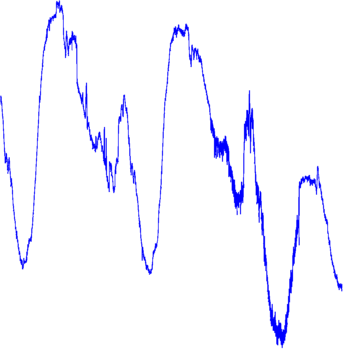1. Start the 1-D Wavelet Analysis Tool.

From the MATLAB prompt, type `waveletAnalyzer`.

The Wavelet Analyzer appears.Click the Wavelet 1-D menu item.

The discrete wavelet analysis tool for 1-D signal data appears.

2. Load a signal.

At the MATLAB command prompt, type

`load leleccum;`
In the Wavelet 1-D tool, select File > Import from Workspace. When the Import from Workspace dialog box appears, select the `leleccum` variable. Click OK to import the electrical consumption signal.

3. Perform a single-level wavelet decomposition.

To start our analysis, let's perform a single-level decomposition using the `db1` wavelet, just as we did using the command-line functions in 1-D Analysis Using the Command Line.

In the upper right portion of the Wavelet 1-D tool, select the `db1` wavelet and single-level decomposition.Click the Analyze button.

After a pause for computation, the tool displays the decomposition.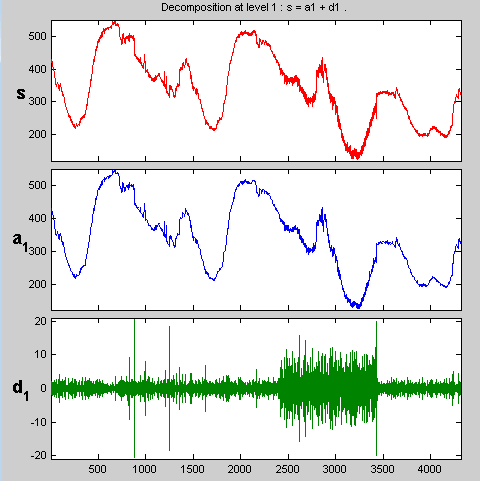4. Zoom in on relevant detail.

One advantage of using the Wavelet Analyzer app is that you can zoom in easily on any part of the signal and examine it in greater detail.

Drag a rubber band box (by holding down the left mouse button) over the portion of the signal you want to magnify. Here, we've selected the noisy part of the original signal.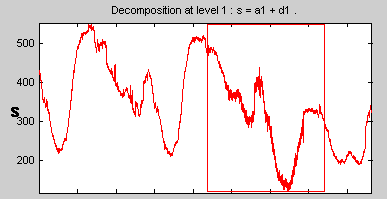Click the X+ button (located at the bottom of the screen) to zoom horizontally.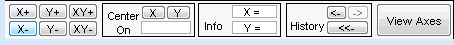The Wavelet 1-D tool zooms all the displayed signals.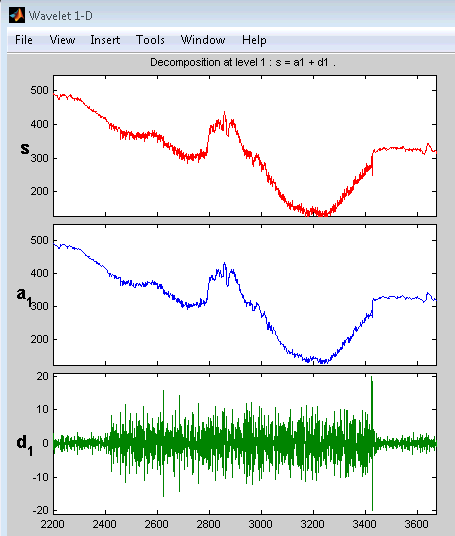The other zoom controls do more or less what you'd expect them to. The X- button, for example, zooms out horizontally. The history function keeps track of all your views of the signal. Return to a previous zoom level by clicking the left arrow button.

5. Perform a multilevel decomposition.

Again, we'll use the graphical tools to emulate what we did in the previous section using command line functions. To perform a level 3 decomposition of the signal using the `db1` wavelet:

Select 3 from the Level menu at the upper right, and then click the Analyze button again.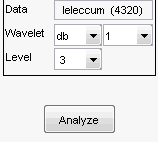After the decomposition is performed, you'll see a new analysis appear in the Wavelet 1-D tool.

Selecting Different Views of the Decomposition

The Display mode menu (middle right) lets you choose different views of the wavelet decomposition.The default display mode is called “Full Decomposition Mode.” Other alternatives include:

• Separate Mode,” which shows the details and the approximations in separate columns.

• Superimpose Mode,” which shows the details on a single plot superimposed in different colors. The approximations are plotted similarly.

• Tree Mode,” which shows the decomposition tree, the original signal, and one additional component of your choice. Click on the decomposition tree to select the signal component you'd like to view.

• Show and Scroll Mode,” which displays three windows. The first shows the original signal superimposed on an approximation you select. The second window shows a detail you select. The third window shows the wavelet coefficients.

• “Show and Scroll Mode (Stem Cfs)” is very similar to the “Show and Scroll Mode” except that it displays, in the third window, the wavelet coefficients as stem plots instead of colored blocks.

You can change the default display mode on a per-session basis. Select the desired mode from the View > Default Display Mode submenu.

Note

The Compression and Denoising windows opened from the Wavelet 1-D tool will inherit the current coefficient visualization attribute (stems or colored blocks).

Depending on which display mode you select, you may have access to additional display options through the More Display Options button.These options include the ability to suppress the display of various components, and to choose whether or not to display the original signal along with the details and approximations.

6. Remove noise from a signal.

The Wavelet Analyzer app features a denoising option with a predefined thresholding strategy. This makes it very easy to remove noise from a signal.

Note

The denoising option is no longer recommended. Use Wavelet Signal Denoiser instead.

Bring up the denoising tool: click the denoise button, located in the middle right of the window, underneath the Analyze button.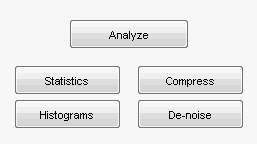The Wavelet 1-D Denoising window appears.

While a number of options are available for fine-tuning the denoising algorithm, we'll accept the defaults of soft fixed form thresholding and unscaled white noise. The `Unscaled white noise` option corresponds to setting the multiplicative threshold input argument `SCAL` of `wden` to `'one'`. Choosing ```Scaled white noise``` corresponds to `'sln'`, and `Non-white noise` corresponds to `'mln'`. For more information, see `wden`.

Continue by clicking the denoise button.

The denoised signal appears superimposed on the original. The tool also plots the wavelet coefficients of both signals. The original detail coefficients appear on the left side of the display. In order to time align decomposition levels across all scales, wavelet coefficients are replicated at each scale to account for the missing time points. Therefore, as the scale becomes coarser, the coefficients assume a staircase-like appearance.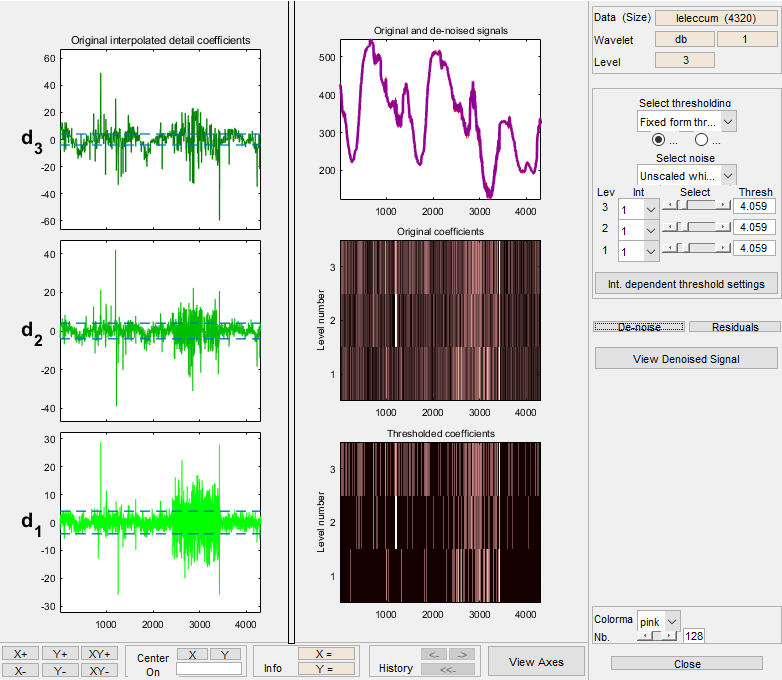Zoom in on the plot of the original and denoised signals for a closer look.

Drag a rubber band box around the pertinent area, and then click the XY+ button.

The denoise window magnifies your view. By default, the original signal is shown in red, and the denoised signal in yellow.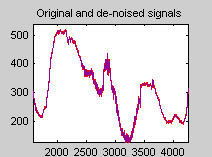Dismiss the Wavelet 1-D Denoising window: click the Close button.

You cannot have the denoise and Compression windows open simultaneously, so close the Wavelet 1-D Denoising window to continue. When the Update Synthesized Signal dialog box appears, click No. If you click Yes, the Synthesized Signal is then available in the Wavelet 1-D main window.

7. Refine the analysis.

The graphical tools make it easy to refine an analysis any time you want to. Up to now, we've looked at a level 3 analysis using `db1`. Let's refine our analysis of the electrical consumption signal using the `db3` wavelet at level 5.

Select 5 from the Level menu at the upper right, and select the `db3` from the Wavelet menu. Click the Analyze button.

8. Compress the signal.

The graphical interface tools feature a compression option with automatic or manual thresholding.Bring up the Compression window: click the Compress button, located in the middle right of the window, underneath the Analyze button.

The Compression window appears.

While you always have the option of choosing by level thresholding, here we'll take advantage of the global thresholding feature for quick and easy compression.

Note

If you want to experiment with manual thresholding, choose the By Level thresholding option from the menu located at the top right of the Wavelet 1-D Compression window. The sliders located below this menu then control the level-dependent thresholds, indicated by yellow dotted lines running horizontally through the graphs on the left of the window. The yellow dotted lines can also be dragged directly using the left mouse button.

Click the Compress button, located at the center right.

After a pause for computation, the electrical consumption signal is redisplayed in red with the compressed version superimposed in yellow. Below, we've zoomed in to get a closer look at the noisy part of the signal.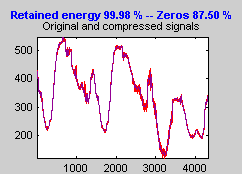You can see that the compression process removed most of the noise, but preserved 99.99% of the energy of the signal.

9. Show the residuals.

From the Wavelet 1-D Compression tool, click the Residuals button. The More on Residuals for Wavelet 1-D Compression window appears.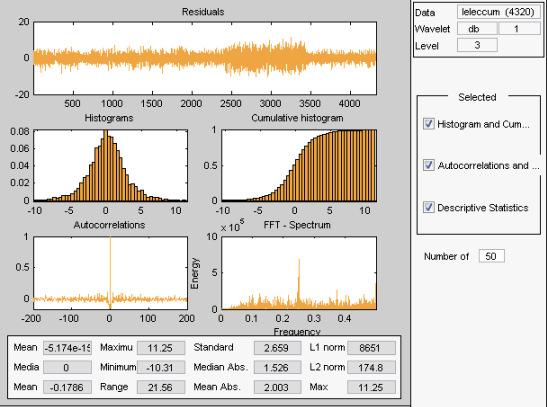Displayed statistics include measures of tendency (mean, mode, median) and dispersion (range, standard deviation). In addition, the tool provides frequency-distribution diagrams (histograms and cumulative histograms), as well as time-series diagrams: autocorrelation function and spectrum. The same feature exists for the Wavelet 1-D Denoising tool.

Dismiss the Wavelet 1-D Compression window: click the Close button. When the Update Synthesized Signal dialog box appears, click No.

10. Show statistics.

You can view a variety of statistics about your signal and its components.

From the Wavelet 1-D tool, click the Statistics button.The Wavelet 1-D Statistics window appears displaying by default statistics on the original signal.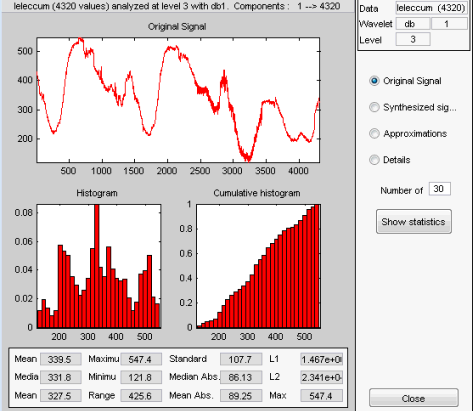Select the synthesized signal or signal component whose statistics you want to examine. Click the appropriate option button, and then click the Show Statistics button. Here, we've chosen to examine the synthesized signal using 100 bins instead of 30, which is the default: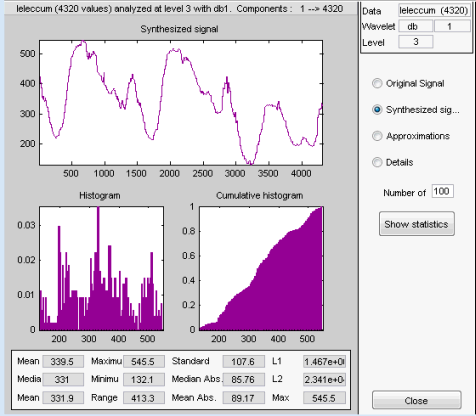Displayed statistics include measures of tendency (mean, mode, median) and dispersion (range, standard deviation).

In addition, the tool provides frequency-distribution diagrams (histograms and cumulative histograms). You can plot these histograms separately using the Histograms button from the Wavelets 1-D window.

Click the Approximation option button. A menu appears from which you choose the level of the approximation you want to examine.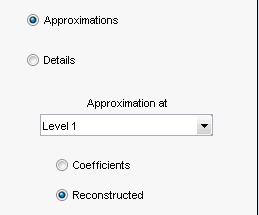Select Level 1 and again click the Show Statistics button. Statistics appear for the level 1 approximation.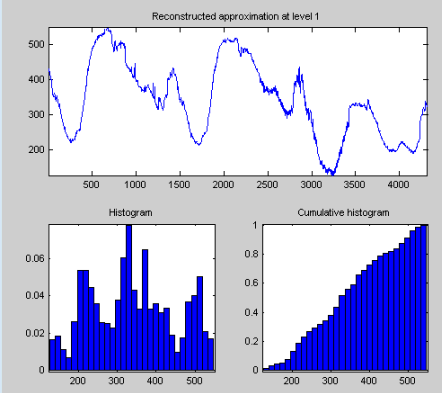### Importing and Exporting Information from the Wavelet Analyzer App

The Wavelet 1-D graphical interface tool lets you import information from and export information to disk and the MATLAB workspace.

#### Saving Information to Disk

You can save synthesized signals, coefficients, and decompositions from the Wavelet 1-D tool to the disk, where the information can be manipulated and later reimported into the graphical tool.Saving Synthesized Signals.  You can process a signal in the Wavelet 1-D tool and then save the processed signal to a MAT-file (with extension `mat` or other).

For example, load the example analysis: File > Example Analysis > Basic Signals > with db3 at level 5 → Sum of sines, and perform a compression or denoising operation on the original signal. When you close the Denoising or Compression window, update the synthesized signal by clicking Yes in the dialog box.

Then, from the Wavelet 1-D tool, select the File > Save > Signal menu option.

A dialog box appears allowing you to select a folder and filename for the MAT-file. For this example, choose the name `synthsig`.

To load the signal into your workspace, simply type

```load synthsig; ```

When the synthesized signal is obtained using any thresholding method except a global one, the saved structure is

```whos ```
NameSizeBytesClass
`synthsig``1x1000``8000``double` array
`thrParams``1x5``580``cell array`
`wname``1x3``6``char array`

The synthesized signal is given by the variable `synthsig`. In addition, the parameters of the denoising or compression process are given by the wavelet name (`wname`) and the level dependent thresholds contained in the `thrParams `variable, which is a cell array of length 5 (same as the level of the decomposition).

For `i` from 1 to 5, `thrParams{i}` contains the lower and upper bounds of the thresholding interval and the threshold value (since interval dependent thresholds are allowed, see 1-D Adaptive Thresholding of Wavelet Coefficients).

For example, for level 1,

```thrParams{1} ans = 1.0e+03 * 0.0010 1.0000 0.0014 ```

When the synthesized signal is obtained using a global thresholding method, the saved structure is

NameSizeBytesClass
`synthsig``1x1000``8000``double array`
`valTHR``1x1``8``double array`
`wname``1x3``6``char array`

where the variable `valTHR` contains the global threshold:

```valTHR valTHR = 1.2922 ```

Saving Discrete Wavelet Transform Coefficients.  The Wavelet 1-D tool lets you save the coefficients of a discrete wavelet transform (DWT) to disk. The toolbox creates a MAT-file in the current folder with a name you choose.

To save the DWT coefficients from the present analysis, use the menu option File > Save > Coefficients.

A dialog box appears that lets you specify a folder and filename for storing the coefficients.

Consider the example analysis:

File > Example Analysis > Basic Signals > with db1 at level 5 → Cantor curve.

After saving the wavelet coefficients to the file `cantor.mat`, load the variables in the workspace:

```load cantor whos```
NameSizeBytesClass
`coefs``1x2190``17520``double array`
`longs``1x7``56``double array`
`thrParams``0x0``0``double array`
`wname``1x3``6``char array`

Variable `coefs` contains the discrete wavelet coefficients. More precisely, in the above example `coefs` is a 1-by-2190 vector of concatenated coefficients, and `longs` is a vector giving the lengths of each component of `coefs`.

Variable `wname` contains the wavelet name and `thrParams` is empty since the synthesized signal does not exist.

Saving Decompositions.  The Wavelet 1-D tool lets you save the entire set of data from a discrete wavelet analysis to disk. The toolbox creates a MAT-file in the current folder with a name you choose, followed by the extension `wa1` (wavelet analysis 1-D).

Open the Wavelet 1-D tool and load the example analysis:

File > Example Analysis > Basic Signals > with db3 at level 5 → Sum of sines

To save the data from this analysis, use the menu option File > Save > Decomposition.

A dialog box appears that lets you specify a folder and filename for storing the decomposition data. Type the name `wdecex1d`.

After saving the decomposition data to the file `wdecex1d.wa1`, load the variables into your workspace:

```load wdecex1d.wa1 -mat whos ```
NameSizeBytesClass
`coefs``1x1023``8184``double array`
`data_name``1x6``12``char array`
`longs``1x7``56``double array`
`thrParams``0x0``0``double array`
`wave_name``1x3``6``char array`

Note

Save options are also available when performing denoising or compression inside the Wavelet 1-D tool. In the Wavelet 1-D Denoising window, you can save denoised signal and decomposition. The same holds true for the Wavelet 1-D Compression window. This way, you can save many different trials from inside the Denoising and Compression windows without going back to the main Wavelet 1-D window during a fine-tuning process.

Note

When saving a synthesized signal, a decomposition or coefficients to a MAT-file, the `mat` file extension is not necessary. You can save approximations individually for each level or save them all at once.

#### Export to Workspace

The Wavelet 1-D tool allows you to export your 1-D wavelet analysis to the MATLAB workspace in a number of formats.

For example, load the example analysis for the `freqbrk` signal.After the wavelet 1-D analysis loads, select ```File —> Export to Workspace```.You have the option to

• `Export Signal` — This option exports the synthesized signal vector.

• `Export Coefficients` — This option exports the vector of wavelet and scaling coefficients, the bookkeeping vector, and the analyzing wavelet in a structure array. The wavelet and scaling coefficient and bookkeeping vectors are identical to the output of `wavedec`.

• `Export Decomposition` — This option is identical to `Export Coefficients` except that it also contains the name of the analyzed signal.

• `Export All Approximations` — This option exports a L-by-N matrix where L is the value of Level and N is the length of the input signal. Each row of the matrix is the projection onto the approximation space at the corresponding level. For example, the first row of the matrix is the projection onto the approximation space at level 1.

• `Export All Details` — This option exports a L-by-N matrix where L is the value of Level and N is the length of the input signal. Each row of the matrix is the projection onto the detail (wavelet) space at the corresponding level. For example, the first row of the matrix is the projection onto the detail space at level 1.

#### Loading Information into the Wavelet 1-D Tool

You can load signals, coefficients, or decompositions into the Wavelet Analyzer app. The information you load may have been previously exported from the app and then manipulated in the workspace, or it may have been information you generated initially from the command line.

In either case, you must observe the strict file formats and data structures used by the Wavelet 1-D tool, or else errors will result when you try to load information.Loading Signals.  To load a signal you've constructed in your MATLAB workspace into the Wavelet 1-D tool, save the signal in a MAT-file (with extension `mat` or other).

For instance, suppose you've designed a signal called `warma` and want to analyze it in the Wavelet 1-D tool.

```save warma warma ```

The workspace variable warma must be a vector.

```sizwarma = size(warma) sizwarma = 1 1000 ```

To load this signal into the Wavelet 1-D tool, use the menu option File > Load > Signal.

A dialog box appears that lets you select the appropriate MAT-file to be loaded.

Note

The first 1-D variable encountered in the file is considered the signal. Variables are inspected in alphabetical order.

Loading Discrete Wavelet Transform Coefficients.  To load discrete wavelet transform coefficients into the Wavelet 1-D tool, you must first save the appropriate data in a MAT-file, which must contain at least the two variables `coefs `and `longs`.

Variable `coefs` must be a vector of DWT coefficients (concatenated for the various levels), and variable `longs` a vector specifying the length of each component of `coefs`, as well as the length of the original signal.After constructing or editing the appropriate data in your workspace, type

```save myfile coefs longs ```

Use the File > Load > Coefficients menu option from the Wavelet 1-D tool to load the data into the graphical tool.

A dialog box appears, allowing you to choose the folder and file in which your data reside.

Loading Decompositions.  To load discrete wavelet transform decomposition data into the Wavelet 1-D graphical interface, you must first save the appropriate data in a MAT-file (with extension `wa1` or other).

The MAT-file contains the following variables.

Variable StatusDescription
`coefs`

Required

Vector of concatenated DWT coefficients

`longs`

Required

Vector specifying lengths of components of `coefs` and of the original signal

`wave_name`

Required

Character vector specifying name of wavelet used for decomposition (e.g., `db3`)

`data_name`

Optional

Character vector specifying name of decomposition

After constructing or editing the appropriate data in your workspace, type

```save myfile coefs longs wave_name ```

Use the File > Load > Decomposition menu option from the Wavelet 1-D tool to load the decomposition data into the graphical tool.

A dialog box appears, allowing you to choose the folder and file in which your data reside.

Note

When loading a signal, a decomposition or coefficients from a MAT-file, the extension of this file is free. The `mat` extension is not necessary.

## SupportGet trial now